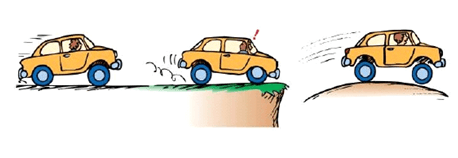Problems
222 - Acceleration

Acceleration

Time Limit: 1 sec

The Problem

Acceleration may be two types. Such as- uniform acceleration and non-uniform acceleration.

If the rate of increase of velocity of a moving body in a particular direction is maintained constant all the time, then the acceleration is said to be uniform.

Conversely, if the rate of increase of velocity changes with time, the acceleration is said to be non-uniform or variable.

The Velocity of a car decreases uniformly from X ms-1 and after T s it becomes Y ms-1. Now he wanted to find the Acceleration of the car.The Input

The input file contains 3 integers X, Y (-100 <=X, Y <=100) and T (0 <= T <= 200).

The Output

For each line of input print the value of Acceleration in a separated line.

Sample Input

25 5 4

Sample Output

-5

Problem Setter: Shaquib Mahmud

Special thanks to Samia Safa Ahmed